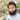# Advance usage of Array.prototype.reduce() in JavaScript

The `reduce()` method takes each element of an array and returns a single value. The method takes a function where you can tell it the type of value you want. When I first came to know to reduce, I had the understanding that it only returns number - most famously, the sum of all the array elements. But it’s more than that!

Let’s get started with the basic usage in case if this is your first introduction, then we’ll move towards the advance usage.

## The basics

In this section, we’ll go through the example of summing up an array of numbers. If you already understand how the reduce works then feel free to skip this section.

``````const numbers = [1, 2, 3, 4];

const sum = numbers.reduce((acc, curr) => acc + curr, 0);

console.log(sum); // 10``````

The `reduce()` takes two arguments - a function (commonly referred to as the reducer function) and an initial value. In the above case, the initial value is `0`.

The reducer function takes four arguments. Only two have been used in the example because those are required. The other two are optional - the index and the array we’re operating on. `acc` and `curr` are the standard conventions to denote the accumulator and the current value respectively.

The second argument of the `reduce()` is the initial value of the accumulator. If this is not provided, the first element of the array will be used. Let’s understand what is happening.

We’re setting the accumulator initial value to `0`. In the first iteration, the current value is `1` which adds up to accumulator value. Hence, `0 + 1` which updates the accumulator value to `1`. In the second iteration, the current value is `2` which adds up to accumulator value. Hence, `1 + 2` which updates the accumulator value to `3`. This process will continue until all the array element is traversed.

What happens with the next iterations? Can you make it to the final value that is `10`?

## Case-1: from array to object

Suppose, we want to turn the `numbers` array to an object with values being the numbers, and keys like `number_1`.

``````/*
Our expaction is -

{
number_1: 1,
number_2: 2,
number_3: 3,
number_4: 4,
}
*/

const numbers = [1, 2, 3, 4];

const numberObj = numbers.reduce((acc, curr) => {
acc[`number_\${curr}`] = curr;

return acc;
}, {});

console.log(numberObj); // meets our expectation!``````

The rule is to first set the initial value of the accumulator to the empty object. Then as we iterate over the array element, we continue to add new properties with values to the object. In the end, return the accumulator which is the resultant object.

Do you want to again return an array? Set the accumulator initial value to `[]`. Let’s do an exercise.

Return an array of `numberObj` i.e. `[{ number_1 : 1},..., {number_4: 4}]`. I know you can do that using `map()`. But for practice, do with reduce. Share your code in the comments!

## Case-2: change the data shape

It is possible to change the shape of the data using the reduce. Let’s look at the below example.

``````/*
we have the following data

{
'x_1': 25,
'x_2': 19,
'x_3': 31,
'y_1': 34,
'y_2': 57,
}

We want to change it to the following shape

{
x: [25, 19, 31],
y: [34, 57],
};
*/

const input = {
x_1: 25,
x_2: 19,
x_3: 31,
y_1: 34,
y_2: 57,
};

const output = Object.entries(input).reduce((acc, curr) => {
const key = curr;

const variable = key.substr(0, key.indexOf('_'));

if (!acc[variable]) {
acc[variable] = [];
}

acc[variable].push(curr);

return acc;
}, {});

console.log(output); // changing the shape done!``````

At first, we get the array of keys and values using Object.entries(). Then, we extract the variable and check that if the variable is already a key in the accumulator object. If so, we push values to the key. Otherwise, we set the value of that key to an empty array. Finally, we return the accumulator.

## Case-3: replacing filter() and map()

Sometimes we need to filter and then map the data. We can achieve the same result using reduce. The micro benefit is to traverse the array once instead of twice.

Let’s take an integers array. We want to keep the even integers and square it.

``````const integers = [1, 2, 3, 4, 5, 6, 7, 8, 9, 10];

const withFilterAndMap = integers
.filter(integer => integer % 2 === 0)
.map(integer => integer ** 2);

console.log(withFilterAndMap); // [ 4, 16, 36, 64, 100 ]

const withReduce = integers.reduce((acc, curr) => {
if (curr % 2 === 0) {
acc.push(curr ** 2);
}

return acc;
}, []);

console.log(withReduce); // [ 4, 16, 36, 64, 100 ]``````

Are you using reduce to do cooler things? Please share in the comment!

### Get the latest post in your inbox!Hi, I'm Md. Kamal Sharif. I'm a senior frontend developer based in Dhaka, Bangladesh.